# 约瑟夫环递归算法（C++）（初学者也能看懂逻辑分析）之美

### 题目：

n个人围成一圈（编号从1到n），从第1个人开始报数，报到m的人出列，从下一个人再重新报数，报到m的人出列，如此下去，直至所有人都出列。求最后一个出列的人的编号。

#include <iostream>
using namespace std;
int josephus(int n, int m)
{
if(n == 1) return 0;
else return ( josephus(n-1,m)+m ) % n;
}

int main()
{
int n, m;
cin >> n >> m;
int result = josephus(n, m);
cout << result+1 << endl;
}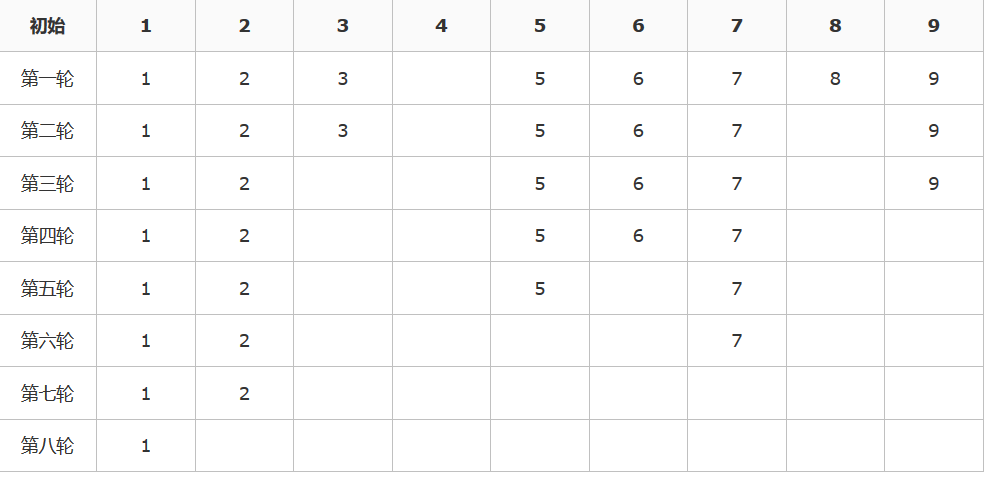### 思路分析1：

1. 既然是递归，那么就是把复杂的问题一步一步分解为最基本、最简单的问题来解决。即把上例的n=9，逐步分解为n=8，n=7，n=6，……，n=2，n=1来解决。
2. 据我们观察，其中输入的变量有两个：一个是人数n，一个是标号数m。所以，假设我们求的递归函数就是f(n,m)f(n,m)。又标号数m是固定的，每次计数的时候都是这个标号。
3. 递归说明，前一个项和后一个项肯定是有关系的，不然递归进行不下去。即f(n,m)f(n,m)与f(n−1,m)f(n−1,m)之间有一定关系

f(n−1,m)之间的关系即可，即找到n=9与n=8，n=8与n=7，……，n=3与n=2，n=2与n=1之间的关系。

### 思路分析2：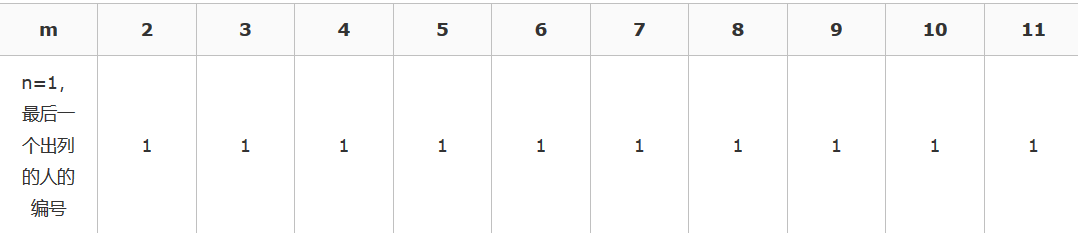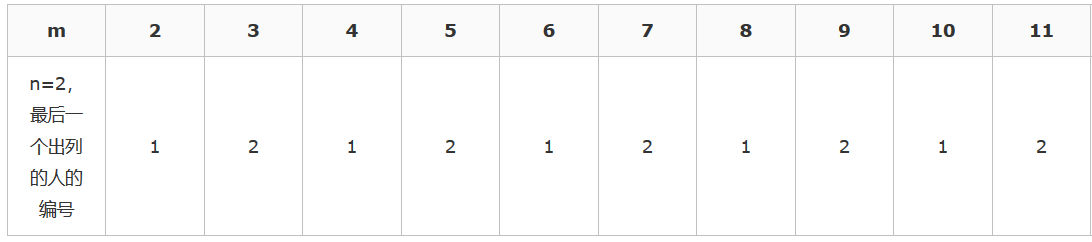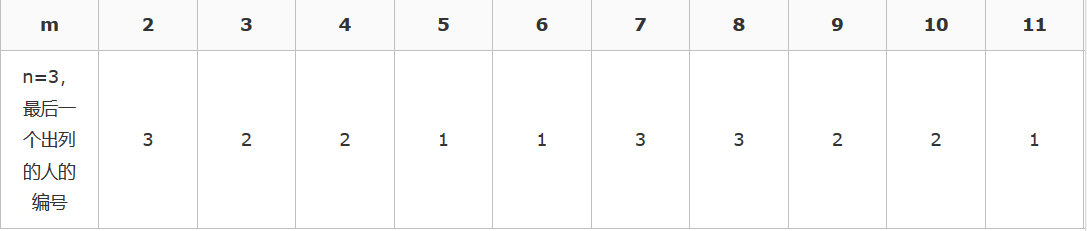f(2,m)了，即每进行一轮，n都减1。

f(3,m)和f(2,m)

f(2,m)之间的递推关系，我们用最笨的方法（穷举法）来找规律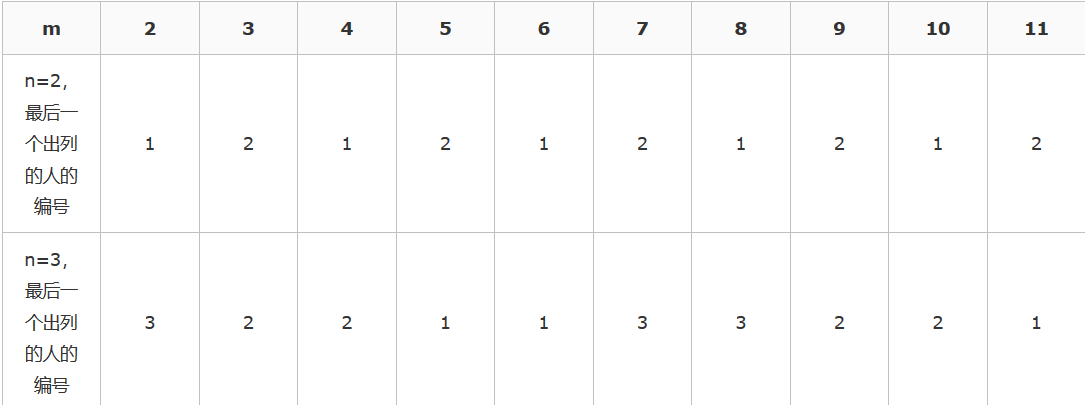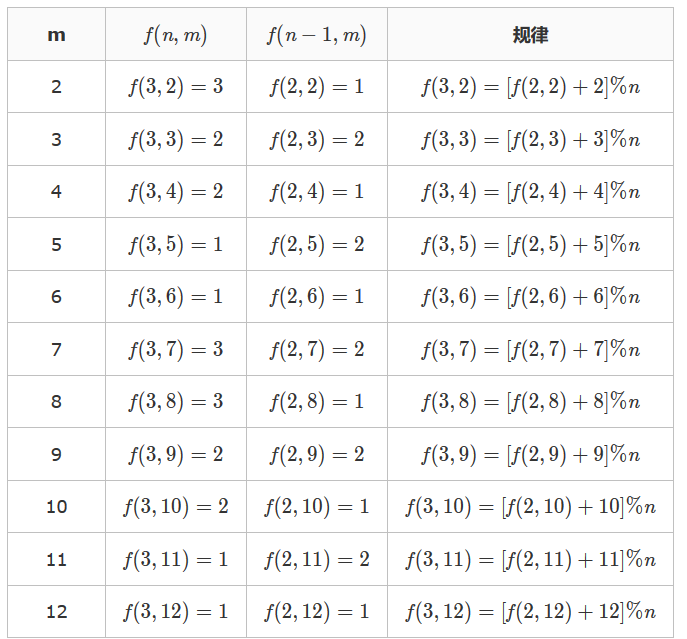f(3,m)之间验证，发现也是正确的。即得出递推关系式。

### 为什么？

f(n,m)=[f(n−1,m)+m]%n，我连找规律都没有找出来，又怎么归纳总结递推关系式？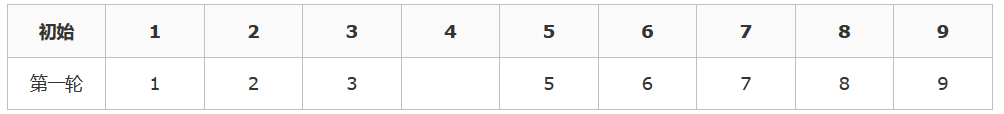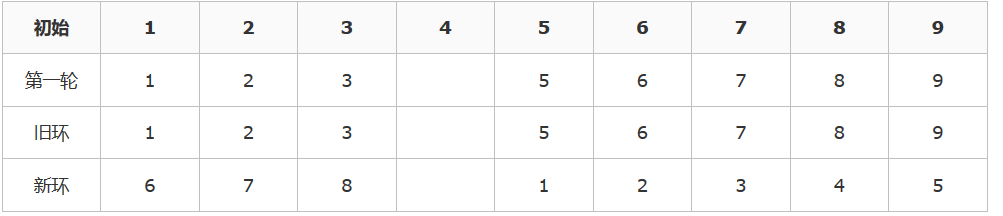(旧的编号)=[(新的编号)+m]%(旧的人数n)

f(n,m)=[f(n−1,m)+m]%n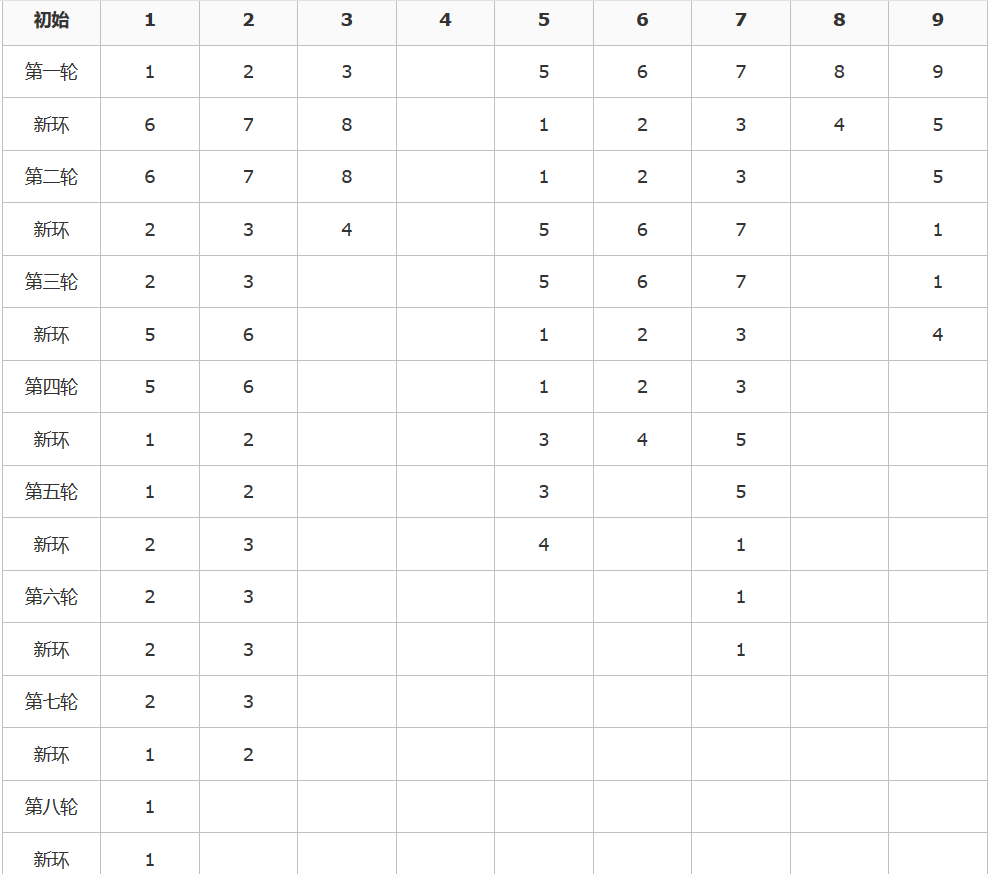#include <iostream>
using namespace std;
int josephus(int n, int m)
{
if(n == 1) return 0;
else return ( josephus(n-1,m)+m ) % n;
}

int main()
{
int n, m;
cin >> n >> m;
int result = josephus(n, m);
cout << result+1 << endl;
}©️2019 CSDN 皮肤主题: 1024 设计师: 上身试试